# Logic Gate Circuits

Subjects:

• Preface
• Logic Gates
• Combinatorial circuits and automotive applications

Preface:
The information processing of modern motor vehicles takes place for the most part, whether or not completely, digitally. The digital information consists of electrical voltages, whereby a yes/no or on/off is formed based on the voltage level. In the interface electronics is located at A/D converter (Analog / Digital) where a sensor voltage is converted to a digital message, which consists of ones and zeros.

In digital electronics we talk about a logic 1 or a logic 0. The voltages are at TTL level (Transistor Transistor Logic).

• Yes or to: logical 1: 5 volt
• No or off: logical 0: 0 volt

Basic electronic circuits on ECUs contain many ICs that make logic circuits. These logic circuits contain logic gates, which can be controlled by the CPU in hardware or software.

Logic gates:
The ALU (Arithmetic Logic Unit, Dutch: arithmetic-logic unit) is the central part of the microprocessor in an ECU. The ALU performs arithmetic (arithmetic) and logic (logical) operations. The ALU also checks where in the memory the next command of the program to be executed is located.

The ALU contains logic gates that are often constructed from silicon semiconductors. The logic gates can perform binary code operations within nanoseconds; a combination of ones and zeros. This gives a command that consists of two options: on or off, conductive or non-conductive. Multiple commands are processed simultaneously in the ALU and work together to form a “word” with 8, 16 or 32 bits, according to the computer architecture. A word is the largest amount of data stored in one data register. This is the amount of data that can be processed by the processor at one time.

The following basic operations take place in an ALU:

• moving one or more bit positions to the left or right (shift)
• perform arithmetic operations on two words, such as addition or completion (add);
• performing logical operations on the data (AND, OR, NOT, NAND, NOR, XOR, XNOR).

In the images below, the ALU is depicted as a symbol (left) and with the IEC symbols translating the operation from A and B (incoming) to R (outgoing).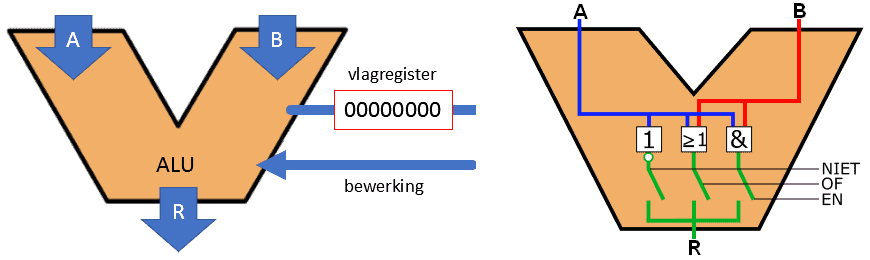The NOT, OR and AND gates that we see in the right ALU are the most common gates used to perform logical operations. There are ports that complement these three basic ports. We will come back to this later on this page. With the NOT, OR and AND gates results of inputs can be preprogrammed. By means of a circuit that puts an answer such as yes/no or true/false to, for example, the parking brake warning lamp, the lamp can be activated on the basis of two inputs.

• is the handbrake actuated?
• is the level of the brake fluid reservoir at the correct level?

The table below shows these three basic ports. We mainly use the English names (AND instead of EN) on this page so as not to create confusion for you as a reader, but both are of course correct. The same goes for the symbols (IEC and ANSI). We use the IEC symbols, but in American literature we mainly see the ANSI symbols. The same applies here: do not mix them up and use one type of symbol.

Below the table an explanation is given what the properties of each port are and the truth table shows with which inputs you get an output of 0 or 1.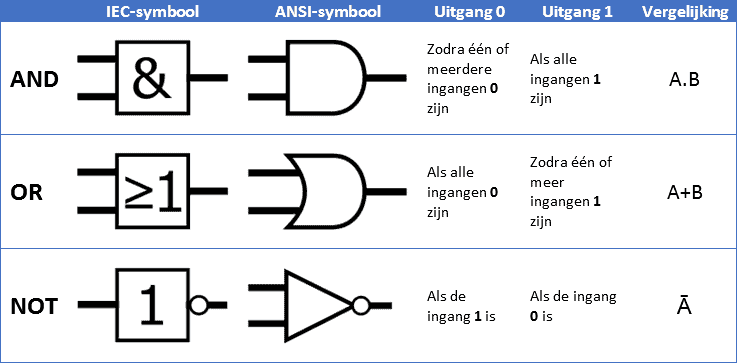Below is the explanation of the three gates with the symbol and the truth table, showing the outputs at different input combinations.

AND gate:
The AND gate (English: AND gate) can have multiple inputs, but always has only one output. In the picture we see the inputs a and b. It is possible to set a 1 or a 0 on both inputs, independently of each other. The output (Q) becomes 1 if both inputs (a and b) are 1. In all other cases the output Q is a 0.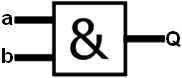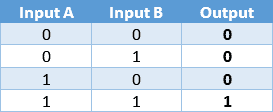• With two inputs to the AND gate (in this case inputs A and B), there are four possible circuits to generate an output. These are shown in the truth table to the right of the AND gate image.
• At four entrances there are 16 possibilities;
• With eight inputs there are even 256 possibilities.

OR gate:
The OR gate can also have multiple inputs, with one output. With an OR gate, the output is 1 if one of the two inputs is a 1, or if both inputs are a 1.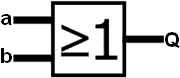NOT gate:
The NOT gate (Dutch: NOT gate) functions as an inverter and has only one input and output. The input signal is inverted: when the input signal is 1, the output signal becomes 0 and vice versa.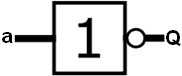In addition to the circuits mentioned (AND, OR and NOT), we also know a number of derived logic circuits. With these circuits we can combine two of the previously discussed circuits into one circuit.NAND gate:
The Not-AND gate is an AND gate followed by a NOT gate. The output is 1 if several inputs have a 1 on them. Only when all inputs have a 1 will the output be a 0. This is the exact opposite of the AND gate discussed earlier.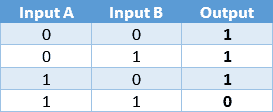NOR gate:
The Not-OR gate is an OR gate followed by a NOT gate. It can have multiple entrances and has only one exit. In this circuit, the output will be 1 only when both inputs are 0.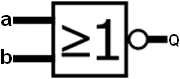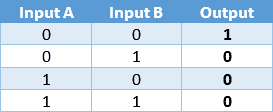XOR gate:
The eXclusive-OR gate is a gate whose output is 1 if only one input is 1. When both inputs have the same logic state, the output becomes a 0. The XOR gate never has more than two inputs.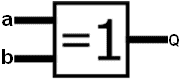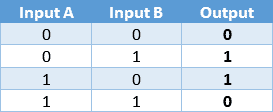XNOR gate:
The eXclusive-OR gate is provided with a NOT gate, which makes it an eXclusive-NOT-OR gate. The output is inverted at the XOR gate.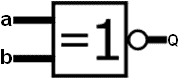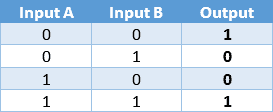For each IC it is important that both a power supply and ground are connected to realize a closed circuit. Also, both ports must be supplied with voltage to prevent a floating measurement. In order for the inputs and outputs to switch properly, pull-up and pull-down resistors are needed. Without these resistors, the gates can remain "active" while not being driven. The ports are then not reliable.

Combinatorial circuits and automotive applications:
The digital ICs can be linked together by connecting the output of one IC to the input of the other IC. With these combinations, circuits can be made with which a desired output combination is obtained for any desired input combination. When several ICs are connected together, we speak of a combinatorial circuit. To get a bit of "feel" with the combinatorial circuits, the following are automotive examples.

Light warning circuit:
A practical example of a combinatorial circuit is that of the light warning. The moment the ignition is switched off and the door is opened while the exterior lights are on, the driver should be warned by means of a buzzer. The AND gate is used for the three input signals. As described in the previous section, all inputs of the AND gate must be 1 to get a 1 on the output and activate the buzzer. If one of the three inputs of the AND gate is a 0, the output will remain 0 and the buzzer will remain muted.

• Light switch: when the switch is switched off, input a shows a 0. When the parking or dipped headlights are switched on, this becomes a 1;
• Ignition lock: when the ignition is switched on, a 1 is placed on input b. A 0 when the ignition is switched off. The NOT gate in this case inverts the 0 into a 1 to obtain the correct signal for the AND gate.
• Door switch: if a door is open, the signal is switched to ground. As with the ignition switch, the 0 must be inverted to a 1 for the AND gate to function properly.error: Alert: Content is protected !!